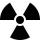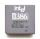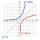# Crystal

Crystal grows every month 1.9 promile of its mass. For how many months to grow a crystal from weight 136 g to 384 g?

Result

n =  546.8

#### Solution:Leave us a comment of example and its solution (i.e. if it is still somewhat unclear...):Be the first to comment!## Next similar examples:A radioactive material loses 10% of its mass each year. What proportion will be left there after n=6 years?
2. PopulationThe town has 65,000 inhabitants. 40 years ago there were 157,000. How many people will live in town in 10 years if the average rate in population is as in previous years?
3. PopulationWhat is the population of the city with 3% annual growth, if in 10 years the city will have 60,000 residents?
4. Six termsFind the first six terms of the sequence a1 = -3, an = 2 * an-1After 548 hours decreases the activity of a radioactive substance to 1/9 of the initial value. What is the half-life of the substance?
6. Computer revolutionWhen we started playing with computers, the first processor, which I remember was the Intel 8080 from 1974, with the performance of 0.5 MIPS. Calculate how much percent a year rose CPU performance when Intel 486DX from 1992 has 54 MIPS. What
7. GP - 8 itemsDetermine the first eight members of a geometric progression if a9=512, q=2
8. LogarithmDetermine the number whose decimal logarithm is -3.8.
9. Tenth memberCalculate the tenth member of geometric sequence when given: a1=1/2 and q=2
10. Theorem proveWe want to prove the sentence: If the natural number n is divisible by six, then n is divisible by three. From what assumption we started?
11. Exponential equationIn the set R solve the equation: ?
12. CoordinateDetermine missing coordinate of the point M [x, 120] of the graph of the function f bv rule: y = 5x
13. Five membersWrite first 5 members geometric sequence and determine whether it is increasing or decreasing: a1 = 3 q = -2
14. Geometric progression 2There is geometric sequence with a1=5.7 and quotient q=-2.5. Calculate a17.
15. Logif ?, what is b?
16. A perineumA perineum string is 10% shorter than its original string. The first string is 24, what is the 9th string or term?
17. Investment1000\$ is invested at 10% compound interest. What factor is the capital multiplied by each year? How much will be there after n=12 years?